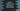# Selection sort in python explanation with example## Selection sort in python explanation with example:

Selection sort is similar to insertion sort. Here, we divide the unsorted list into two parts. One part is sorted and another part is unsorted. It searches for the smallest element in the unsorted list and place it in the correct position in the sorted list.

So, in each iteration of the unsorted part, it picks the smallest element from the unsorted part and inserted into the sorted part.

### Algorithm for selection sort:

We will follow the below algorithm for selection sort:

• Start the sorted sublist from left of the unsorted list.
• At first, the sorted sublist is empty and unsorted sublist includes all other elements of the list.
• In each iteration, find the smallest element in the unsorted list and swap it with the leftmost element of the unsorted list.
• After the swap, increase the size of the sorted list by 1. Also, decrease the size of the unsorted list by 1.
• After each iteration, one item will be inserted to the sorted list and it will increase its size by 1. At the end, we will have only one sorted list holding all elements of the original list.

Below is the algorithm:

``````selection_sort(array):
size = len(array)
loop from i = 0 to i = n:
current_min_index = i
loop from j = i+1 to j = n:
if array[current_min_index] > array[j]:
current_min_index = j

swap(array[i], array[current_min_index])``````

### Example of selection sort:

Let’s take a look at the below example:

In this example,

• Green area is the sorted subarray and red area is the unsorted subarray.
• On each iteration, we are finding the smallest element from the unsorted subarray and swapping it with the first element of the unsorted array. Then, we are incrementing the length of the sorted subarray by 1.

### Python program:

Below is the complete python program that implements selection sort:

``````def swap(arr, i, j):
temp = arr[i]
arr[i] = arr[j]
arr[j] = temp

def selection_sort(arr):
size = len(arr)

for i in range(size):
current_min_index = i

for j in range(i+1, size):
if arr[current_min_index] > arr[j]:
current_min_index = j

if i != current_min_index:
swap(arr, i, current_min_index)

return arr

if __name__ == '__main__':
arr = [1, 5, 6, 12, 4, 8, 19, 99, 20, 77, 66, 34, 55]
print("Given array : {}".format(arr))
print("Array after selection sort : {}".format(selection_sort(arr)))``````

Here,

• selection_sort is used to sort the given array using selection sort.
• swap swaps two items in an array.
• It uses the same algorithm that we discussed before.

If you run this program, it will print the below output:

``````Given array : [1, 5, 6, 12, 4, 8, 19, 99, 20, 77, 66, 34, 55]
Array after selection sort : [1, 4, 5, 6, 8, 12, 19, 20, 34, 55, 66, 77, 99]``````